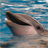# Script No longer is working

609
0
10-21-2011 12:35 PMNew Contributor
I have used this script for quite some time to figure a variable of the soils layer.  I don't recall if I have used it since I upgraded to ArcMap 10.  I'm looking for a helping hand as I don't know anything about python.

Here is the script that I use in the field calculator

```IF RIGHT ([LCC],1) = "E" THEN
X = 10
ELSEIF [LCC] = "2W" THEN
X = 70
ELSEIF RIGHT ([LCC],1) = "W" THEN
X = 100
ELSEIF RIGHT ([LCC],1) = "S" AND [DRNCLSCD] <45 THEN
X = 10
ELSEIF RIGHT ([LCC],1) = "S" AND [DRNCLSCD] <60 THEN
X = 40
ELSEIF RIGHT ([LCC],1) = "S" AND [DRNCLSCD] <70 THEN
X = 70
ELSEIF RIGHT ([LCC],1) = "S" AND [DRNCLSCD] =70 THEN
X = 100
ELSEIF [LCC] = "1"THEN
X = 40
ELSE
X = 0
ENDIF
__esri_field_calculator_splitter__
x```

I am using Windows 7 Profession, 64bit

Any help would be greatly appreciated.  I am still a noob when it comes to most of this.